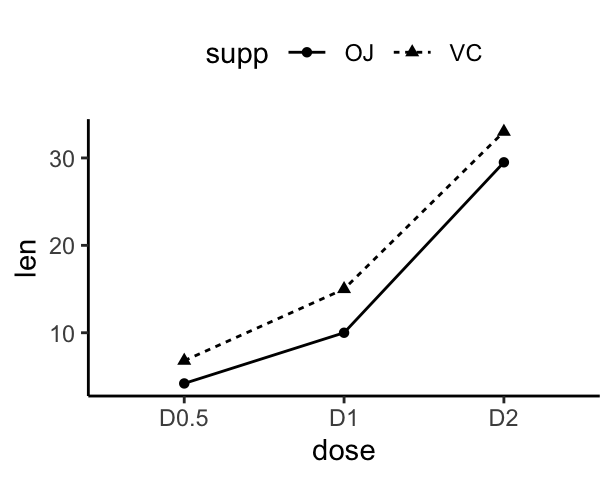# Data Visualization using GGPlot2

## GGPlot Line Plot

In a line plot, observations are ordered by x value and connected by a line.

x value (for x axis) can be :

• date : for a time series data
• texts
• discrete numeric values
• continuous numeric values

This article describes how to create a line plot using the ggplot2 R package

You will learn how to:

• Create basic and grouped line plots
• Add points to a line plot
• Change the line types and colors by group

Contents:

#### Related Book

GGPlot2 Essentials for Great Data Visualization in R

## Key R functions

• Key functions:
• geom_path() connects the observations in the order in which they appear in the data.
• geom_line() connects them in order of the variable on the x axis.
• geom_step() creates a stairstep plot, highlighting exactly when changes occur.
• Key arguments to customize the plot: alpha, color, linetype and size

## Data preparation

We’ll create two data frames derived from the ToothGrowth datasets.

df <- data.frame(dose=c("D0.5", "D1", "D2"),
len=c(4.2, 10, 29.5))

head(df, 4)
##   dose  len
## 1 D0.5  4.2
## 2   D1 10.0
## 3   D2 29.5
df2 <- data.frame(supp=rep(c("VC", "OJ"), each=3),
dose=rep(c("D0.5", "D1", "D2"),2),
len=c(6.8, 15, 33, 4.2, 10, 29.5))

head(df2, 4)
##   supp dose  len
## 1   VC D0.5  6.8
## 2   VC   D1 15.0
## 3   VC   D2 33.0
## 4   OJ D0.5  4.2
• len: Tooth length
• dose: Dose in milligrams (0.5, 1, 2)
• supp: Supplement type (VC or OJ)

Load the ggplot2 package and set the default theme to theme_classic() with the legend at the top of the plot:

library(ggplot2)
theme_set(
theme_classic() +
theme(legend.position = "top")
)

## Basic line plots

p <- ggplot(data = df, aes(x = dose, y = len, group = 1))
# Basic line plot with points
p + geom_line() + geom_point()

# Change line type and color
p + geom_line(linetype = "dashed", color = "steelblue")+
geom_point(color = "steelblue")

# Use geom_step()
p + geom_step() + geom_point()Note that, the group aesthetic determines which cases are connected together.

## Line plot with multiple groups

In the graphs below, line types and point shapes are controlled automatically by the levels of the variable supp:

p <- ggplot(df2, aes(x = dose, y = len, group = supp))
# Change line types and point shapes by groups
p + geom_line(aes(linetype = supp)) +
geom_point(aes(shape = supp))

# Change line types, point shapes and colors
# Change color manually: custom color
p + geom_line(aes(linetype = supp, color = supp))+
geom_point(aes(shape = supp, color = supp)) +
scale_color_manual(values=c("#999999", "#E69F00"))## Line plot with a numeric x-axis

If the variable on x-axis is numeric, it can be useful to treat it as a continuous or a factor variable depending on what you want to do:

# Create some data
df3 <- data.frame(supp=rep(c("VC", "OJ"), each=3),
dose=rep(c("0.5", "1", "2"),2),
len=c(6.8, 15, 33, 4.2, 10, 29.5))
head(df3)
##   supp dose  len
## 1   VC  0.5  6.8
## 2   VC    1 15.0
## 3   VC    2 33.0
## 4   OJ  0.5  4.2
## 5   OJ    1 10.0
## 6   OJ    2 29.5
# x axis treated as continuous variable
df3$dose <- as.numeric(as.vector(df3$dose))
ggplot(data = df3, aes(x = dose, y = len, group = supp, color = supp)) +
geom_line() + geom_point()

# Axis treated as discrete variable
df3$dose<-as.factor(df3$dose)
ggplot(data=df3, aes(x = dose, y = len, group = supp, color = supp)) +
geom_line() + geom_point()## Line plot with dates on x-axis: Time series

economics time series data sets are used :

head(economics)
## # A tibble: 6 x 6
##   date         pce    pop psavert uempmed unemploy
##   <date>     <dbl>  <int>   <dbl>   <dbl>    <int>
## 1 1967-07-01  507. 198712    12.5     4.5     2944
## 2 1967-08-01  510. 198911    12.5     4.7     2945
## 3 1967-09-01  516. 199113    11.7     4.6     2958
## 4 1967-10-01  513. 199311    12.5     4.9     3143
## 5 1967-11-01  518. 199498    12.5     4.7     3066
## 6 1967-12-01  526. 199657    12.1     4.8     3018

Plots :

# Basic line plot
ggplot(data=economics, aes(x = date, y = pop))+
geom_line()

# Plot a subset of the data
ss <- subset(economics, date > as.Date("2006-1-1"))
ggplot(data = ss, aes(x = date, y = pop)) + geom_line()Change line size :

ggplot(data = economics, aes(x = date, y = pop)) +
geom_line(aes(size = unemploy/pop))Plot multiple time series data:

ggplot(economics, aes(x=date)) +
geom_line(aes(y = psavert), color = "darkred") +
geom_line(aes(y = uempmed), color="steelblue", linetype="twodash")# Area plot
ggplot(economics, aes(x=date)) +
geom_area(aes(y = psavert), fill = "#999999",
color = "#999999", alpha=0.5) +
geom_area(aes(y = uempmed), fill = "#E69F00",
color = "#E69F00",  alpha=0.5)## Conclusion

This article shows how to create line plots using the ggplot2 package.